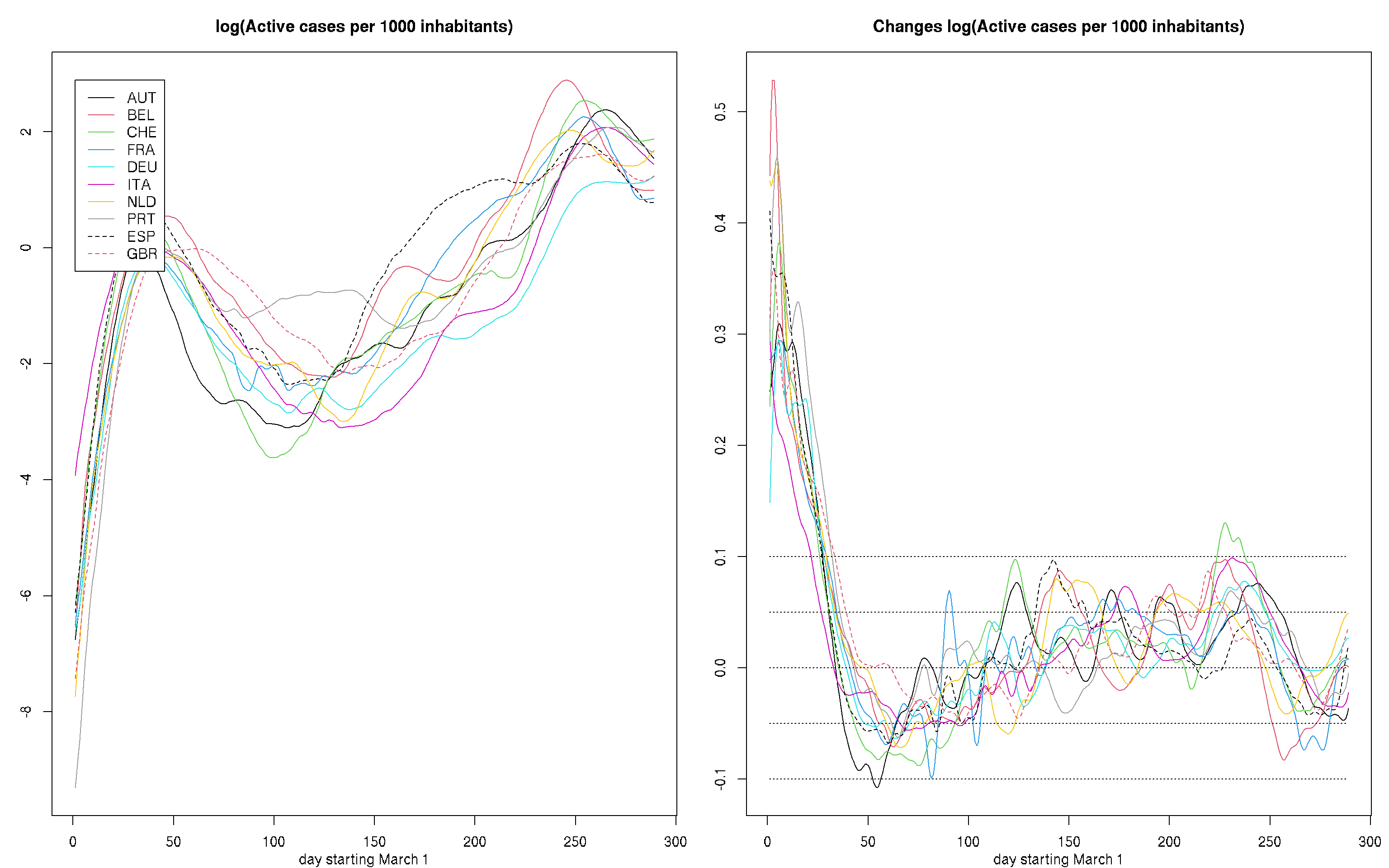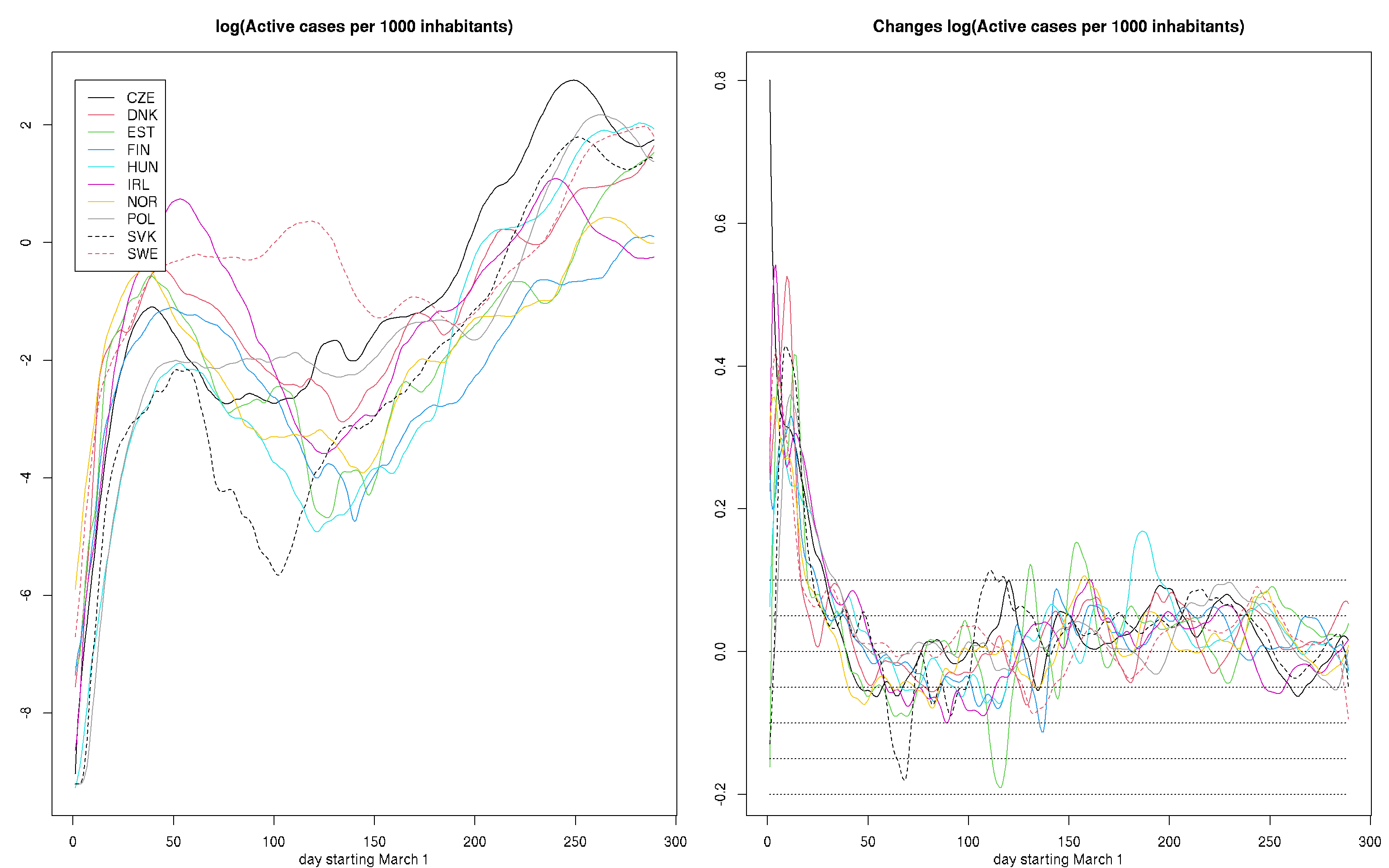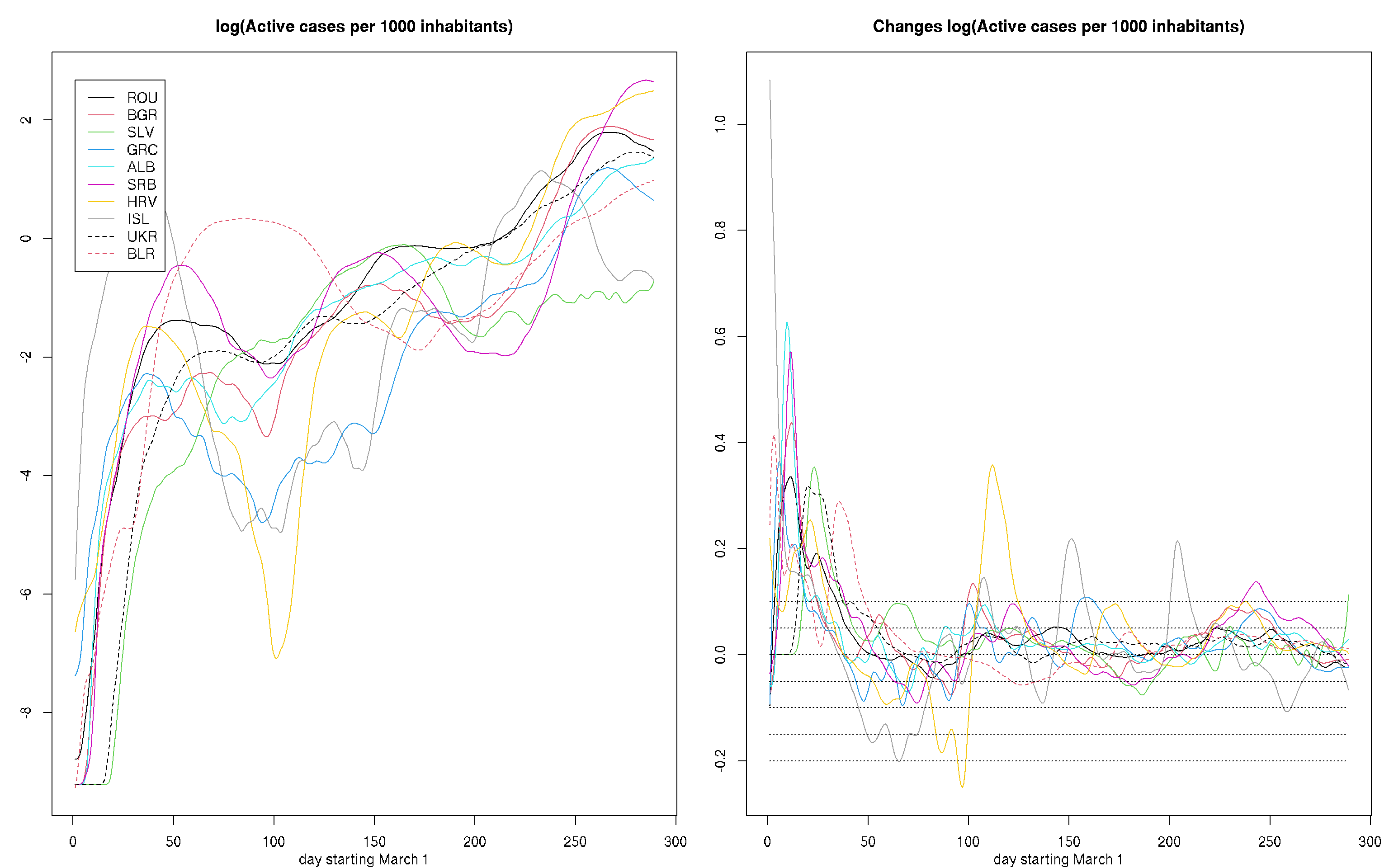## General remarks

This analysis is based on the daily updated numbers of confirmed infections provided by the European Centre for Disease Prevention and Control.

``````#library(XLConnect)1
data <- read.csv("https://opendata.ecdc.europa.eu/covid19/casedistribution/csv", na.strings = "", fileEncoding = "UTF-8-BOM")
countries <- c("AUS","AUT","BEL","BRA","CAN","CZE","DNK","EST","CHE","FIN","FRA","DEU","HUN","ISL","IRL","ISR","ITA","JPN","NLD","NZL","NOR","POL","PRT","RUS","SVK","KOR","ESP","SWE","TUR","GBR","USA","ARG","CHL","URY","CUB","PER","MEX","ECU","ZAF","SGP","UKR","BLR","ROU","PAN","IRN","KWT","ARE","EGY","SAU","QAT","BGR","SLV","GRC","ALB","SRB","HRV","BOL","COL","ETH","IND")
data <- data[data\$countryterritoryCode%in%countries,c(1:6,9:11)]
nentries <- dim(data)
udates <- unique(data[,1])
ndates <- length(udates)
udates <- udates[ndates:1]
nc <- length(countries)
pop <- numeric(nc)
cases <- deaths <- matrix(0,ndates,nc)
for(i in 1:nentries){
j <- (1:ndates)[udates==data\$dateRep[i]]
k <- (1:nc)[countries==data\$countryterritoryCode[i]]
cases[j,k] <- data\$cases[i]
deaths[j,k] <- data\$deaths[i]
pop[k] <- data\$popData2019[i]/1000
}
pop <- 4246.44 # data value for Panama  is wrong
cases[186,30] <- 0 #UK
cases1 <- apply(cases,2,cumsum)
rate <- sweep(cases1,2,pop,"/")[-(1:61),] # remove data before March 1
rate[rate < 1e-4] <- 1e-4
diffrate <- apply(rate,2,diff)``````

For modeling we use local polynomial regression as implemented in function locpoly in R package KernSmooth. We employ a Gaussian kernel with bandwidth (kernel standard deviation) 1.5 days for estimating rates and 2.5 for estimating derivatives. Polynomial orders are 1 and 2, respectiviley, to minimize boundary bias. dates are in days after March 1.

Please note that the estimated curves for small number of reported cases carry a high variability. This is especially due for early dates and states with low population (ISL, EST). Problems in the data like underreporting and reporting bias around weekends are not modeled.

## Active cases

Active cases are here defined as cases that were reported within the last 14 days. This is more conservative than the rule used, e.g., by RKI (corresponding to approxi3mately the last 11 days) and was chosen to minimize effects due to a weekly cycly of reporting biases observed in the data. 1

``````ieurope1 <- c(2,3,9,11,12,17,19,23,27,30)
ieurope2 <- c(6,7,8,10,13,15,21,22,25,28)
ieurope3 <- c(43,51:56,14,41,42)
iworld1 <- c(1,5,16,18,20,24,26,29,31,60)
iworld2 <- c(4,32:40)
iworld3 <- c(44:50,57:59)``````
``````bw <- 1.5
dbw <- 2.5
dates <- 1:(ndates-61) # exclude info before March 1
inc <- 14
rlcurves <- dlcurves <- list(NULL)
rlylim <- dlylim <- NULL
for (i in 1:nc) {
rlcurves[[i]]<- locpoly(dates,log(pmax(1e-4,diff(c(rep(0,inc),rate[,i]),inc))),bandwidth=bw,degree=1)
rlylim <- range(rlylim,rlcurves[[i]]\$y)
dlcurves[[i]] <- locpoly(dates,log(pmax(1e-4,diff(c(rep(0,inc),rate[,i]),inc))),bandwidth=dbw,drv=1,degree=2)
dlylim <- range(dlylim,dlcurves[[i]]\$y)
}
rlylim1 <- dlylim1 <- NULL
for(i in ieurope1){
rlylim1 <- range(rlylim1,rlcurves[[i]]\$y)
dlylim1 <- range(dlylim1,dlcurves[[i]]\$y)
}
rlylim2 <- dlylim2 <- NULL
for(i in ieurope2){
rlylim2 <- range(rlylim2,rlcurves[[i]]\$y)
dlylim2 <- range(dlylim2,dlcurves[[i]]\$y)
}
rlylim2a <- dlylim2a <- NULL
for(i in ieurope3){
rlylim2a <- range(rlylim2a,rlcurves[[i]]\$y)
dlylim2a <- range(dlylim2a,dlcurves[[i]]\$y)
}
rlylim3 <- dlylim3 <- NULL
for(i in iworld1){
rlylim3 <- range(rlylim3,rlcurves[[i]]\$y)
dlylim3 <- range(dlylim3,dlcurves[[i]]\$y)
}
rlylim4 <- dlylim4 <- NULL
for(i in iworld2){
rlylim4 <- range(rlylim4,rlcurves[[i]]\$y)
dlylim4 <- range(dlylim4,dlcurves[[i]]\$y)
}
rlylim5 <- dlylim5 <- NULL
for(i in iworld3){
rlylim5 <- range(rlylim5,rlcurves[[i]]\$y)
dlylim5 <- range(dlylim5,dlcurves[[i]]\$y)
}``````

The left plot provides estimates of the logarithmic rate of of active cases while the right hand site displays its first derivative.

``````par(mfrow=c(1,2),mar=c(3,3,3,1),mgp=c(2,1,0))
plot(dates,rate[,1],ylim = rlylim1,type="n",xlab="day starting March 1",ylab="")
for(i in 1:10)
lines(rlcurves[[ieurope1[i]]]\$x,rlcurves[[ieurope1[i]]]\$y,col=i,lty=1+(i-1)%/%8)
legend(1,rlylim1,countries[ieurope1],col=c(1:10),lty=c(1+(0:9)%/%8),lwd=rep(1,10))
title("log(Active cases per 1000 inhabitants)")

plot(dates,c(0,diffrate[,1]),ylim = dlylim1,type="n",xlab="day starting March 1",ylab="")
for(i in 1:10) lines(dlcurves[[ieurope1[i]]]\$x,dlcurves[[ieurope1[i]]]\$y,col=i,lty=1+(i-1)%/%8)
title("Changes log(Active cases per 1000 inhabitants)")
for(lvl in seq(-.2,.1,.05)) lines(range(dates),c(lvl,lvl),lty=3)````````````par(mfrow=c(1,2),mar=c(3,3,3,1),mgp=c(2,1,0))
plot(dates,rate[,1],ylim= rlylim2,type="n",xlab="day starting March 1",ylab="")
for(i in 1:10)
lines(rlcurves[[ieurope2[i]]]\$x,rlcurves[[ieurope2[i]]]\$y,col=i,lty=1+(i-1)%/%8)
legend(1,rlylim2,countries[ieurope2],col=c(1:10),lty=c(1+(0:9)%/%8),lwd=rep(1,10))
title("log(Active cases per 1000 inhabitants)")

plot(dates,c(0,diffrate[,1]),ylim = dlylim2,type="n",xlab="day starting March 1",ylab="")
for(i in 1:10) lines(dlcurves[[ieurope2[i]]]\$x,dlcurves[[ieurope2[i]]]\$y,col=i,lty=1+(i-1)%/%8)
title("Changes log(Active cases per 1000 inhabitants)")
for(lvl in seq(-.2,.1,.05)) lines(range(dates),c(lvl,lvl),lty=3)````````````par(mfrow=c(1,2),mar=c(3,3,3,1),mgp=c(2,1,0))
plot(dates,rate[,1],ylim= rlylim2a,type="n",xlab="day starting March 1",ylab="")
for(i in 1:10)
lines(rlcurves[[ieurope3[i]]]\$x,rlcurves[[ieurope3[i]]]\$y,col=i,lty=1+(i-1)%/%8)
legend(1,rlylim2a,countries[ieurope3],col=c(1:10),lty=c(1+(0:9)%/%8),lwd=rep(1,10))
title("log(Active cases per 1000 inhabitants)")

plot(dates,c(0,diffrate[,1]),ylim = dlylim2a,type="n",xlab="day starting March 1",ylab="")
for(i in 1:10) lines(dlcurves[[ieurope3[i]]]\$x,dlcurves[[ieurope3[i]]]\$y,col=i,lty=1+(i-1)%/%8)
title("Changes log(Active cases per 1000 inhabitants)")
for(lvl in seq(-.2,.1,.05)) lines(range(dates),c(lvl,lvl),lty=3)````````````par(mfrow=c(1,2),mar=c(3,3,3,1),mgp=c(2,1,0))
plot(dates,rate[,1],ylim = rlylim3,type="n",xlab="day starting March 1",ylab="")
for(i in 1:10)
lines(rlcurves[[iworld1[i]]]\$x,rlcurves[[iworld1[i]]]\$y,col=i,lty=1+(i-1)%/%8)
legend(1,rlylim3,countries[iworld1],col=c(1:10),lty=c(1+(0:9)%/%8),lwd=rep(1,10))
title("log(Active cases per 1000 inhabitants)")

plot(dates,c(0,diffrate[,1]),ylim = dlylim3,type="n",xlab="day starting March 1",ylab="")
for(i in 1:10) lines(dlcurves[[iworld1[i]]]\$x,dlcurves[[iworld1[i]]]\$y,col=i,lty=1+(i-1)%/%8)
title("Changes log1(Active cases per 1000 inhabitants)")
for(lvl in seq(-.2,.1,.05)) lines(range(dates),c(lvl,lvl),lty=3)``````